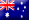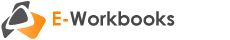# Introductory StatisticsThe purpose of this e-workbook is to introduce students to the tools and methods of statistical analysis. Practical business applications are emphasised throughout the resource. Students are guided through the process of collecting data, developing tools, analysing data, making and testing inferences and modelling.

## Part 1 - Collecting data

#### Collection and presentation of data

• Data collection and classification
• Presenting categorical data
• Presenting numerical data
• Contingency tables
• Scatter diagrams
• Time-series plots

## Part 2 - Developing tools

#### Numerical descriptive measures

• Measures of a population
• Measures of centre
• Measures of variation
• Data analysis
• Measures of linear relation

#### Probability

• Introductory probability
• Conditional probability
• Bayes’ theorem
• Enumeration

#### Discrete probability distributions

• Discrete random variables
• An application to finance: portfolio theory
• The binomial distribution
• The Poisson distribution
• The hypergeometric distribution

#### Continuous probability distributions

• The uniform distribution
• The normal distribution
• Determination of normality
• Normal approximation to the binomial distribution
• The exponential distribution

## Part 3 - Analysis, inference and testing

#### Sampling distributions

• The distribution of sample means
• The distribution of sample proportions
• Sampling techniques
• Survey credibility

#### Confidence intervals

• Confidence interval for the mean
• Confidence interval for the proportion
• Required sample size
• An application to business: auditing

#### Hypothesis testing

• Concepts of hypothesis testing
• The power of a hypothesis test
• z test of hypotheses for the mean
• t test of hypotheses for the mean
• z test of hypotheses for the proportion
• One-tail tests

#### Comparing populations

• Comparing two means
• z test for the difference between two proportions
• F test for the difference between two variances
• One-way analysis of variance

#### Chi-square tests

• Comparing two proportions
• Comparing more than two proportions
• Chi-square test for independence
• Chi-square test for a population variance
• Chi-square goodness-of-fit tests

## Part 4 - Modeling

#### Simple linear regression

• Constructing the linear regression equation
• Measuring error in linear regression
• The assumptions of linear regression
• Testing the model
• Approximating the mean value
• Constructing a prediction interval

#### Multiple regression

• Constructing the multiple regression model
• The assumptions of multiple linear regression
• F test of a multiple regression model
• t test of the slope coefficients
• Partial F test of an independent variable
• Regression for qualitative data

#### Time-series and index numbers

• Time-series and smoothing techniques
• Trend-fitting and forecasting
• Selecting a model for a time-series
• Forecasting for seasonal data
• Index numbers